#Rokid webhook 指南 手把手教你做个懒人

0
0
01. 云栖社区>
2. Rokid>
3. 博客>
4. 正文

## Rokid webhook 指南 手把手教你做个懒人

《如何使用 Rokid 的 webhook 功能》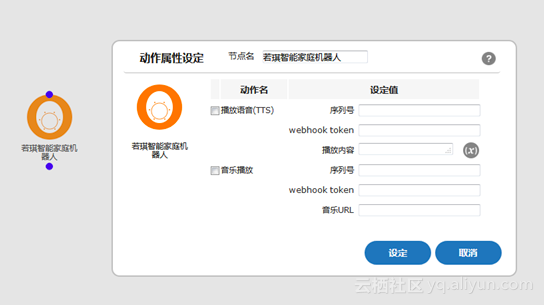### 基本概念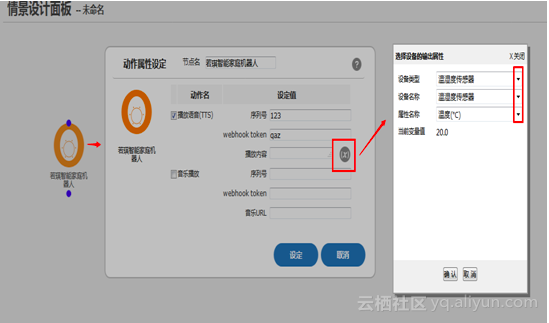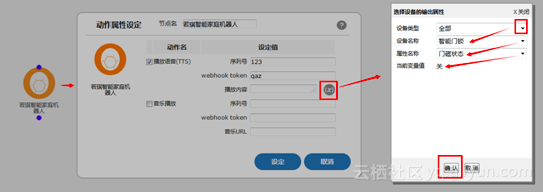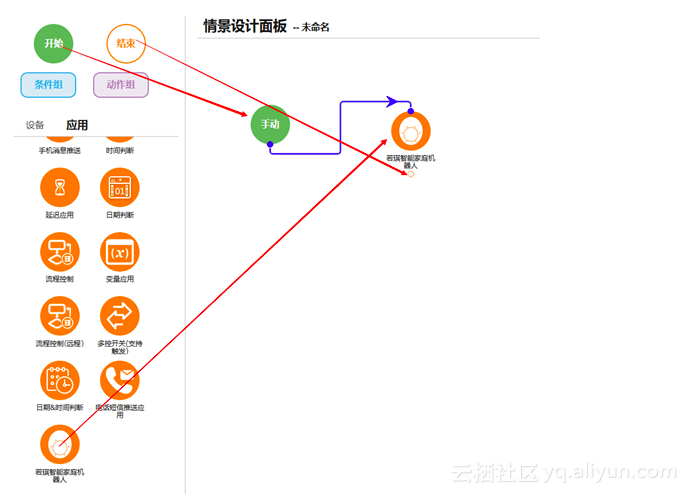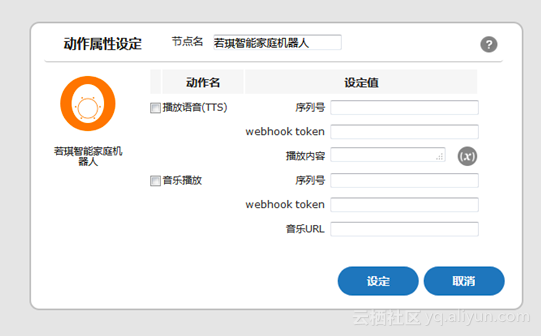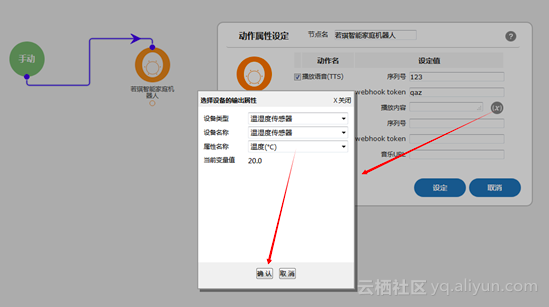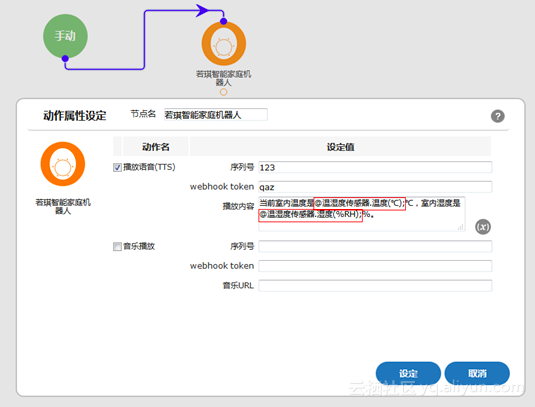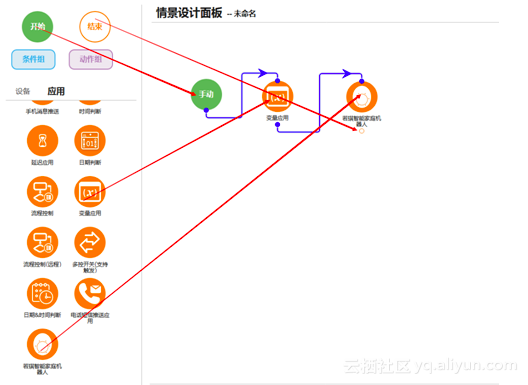.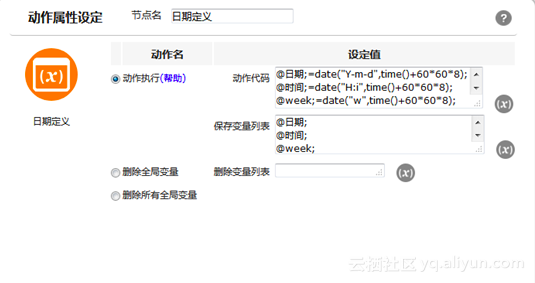@日期;=date("Y-m-d",time()+60*60*8);

@时间;=date("H:i",time()+60*60*8);

@week;=date("w",time()+60*60*8);

@日期;=date("Y-m-d",time()+60*60*8); 

@日期;=date("m-d",time()+60*60*8);

@月;=date("m",time()+60*60*8);

@日;=date("d",time()+60*60*8);

@week;=date("w",time()+60*60*8);

@星期;=$wk[date("w",time()+60*60*8)];$wk=array("日","一","二","三","四","五","六");

@日期;=date("Y-m-d",time()+60*60*8);

@月;=date("m",time()+60*60*8);

@日;=date("d",time()+60*60*8);

@时间;=date("H:i",time()+60*60*8);

$wk=array("日","一","二","三","四","五","六"); @星期;=$wk[date("w",time()+60*60*8)];

+ 关注